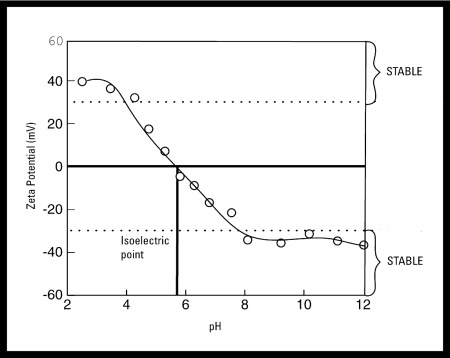The Influence of Zeta Potential Zeta Potential and pH The most important factor that affects zeta potential is pH A zeta potential value quoted without a definition of it's environment (pH, ionic strength, concentration of any additives) is a meaningless number Imagine a particle in suspension with a negative zeta potential If more alkali is added to this suspension then the particles tend to acquire more negative charge If acid is added to this suspension then a point will be reached where the charge will be neutralized Further addition of acid will cause a build up of positive charge In general, a zeta potential versus pH curve will be positive at low pH and lower or negative at high pH There may be a point where the curve passes through zero zeta potential This point is called the isoelectric point and is very important from a practical consideration It is normally the point where the colloidal system is least stableIn the above example it can be seen that if the dispersion pH is below 4 or above 8 there is sufficient charge to confer stability. However if the pH of the system is between 4 and 8 the dispersion may be unstable. This is most likely to be the case at around pH 6 (the isoelectric point)